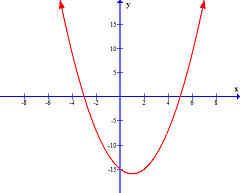# Fundamental Theorem of Algebra: Explanation and Example

Instructions:

Choose an answer and hit 'next'. You will receive your score and answers at the end.

question 1 of 3

### According to the Fundamental Theorem of Algebra, the polynomial function f(x) = x^3 + 5x^2 - x - 5 has how many complex solutions?

Create Your Account To Take This Quiz

As a member, you'll also get unlimited access to over 75,000 lessons in math, English, science, history, and more. Plus, get practice tests, quizzes, and personalized coaching to help you succeed.

Try it risk-free for 30 days. Cancel anytime.

### 2. The following graph is of a polynomial function of degree 2. Are the solutions of this function real or imaginary and why?Create your account to access this entire worksheet
A Premium account gives you access to all lesson, practice exams, quizzes & worksheets
Quizzes, practice exams & worksheets
Certificate of Completion
Create an account to get started

Learn how to find the number of solutions in a polynomial function with this helpful, easy-to-understand worksheet and quiz set focused on the fundamental theorem of algebra. Our quiz will gauge your ability to solve problems involving polynomial functions and graphs illustrating a function's solutions.

## Quiz & Worksheet Goals

These materials on the fundamental theorem of algebra will teach you how to:

• Define the fundamental theorem and the term complex solutions
• Find the number of solutions for a polynomial function
• Graph polynomial functions
• Determine when a function has repeated solutions
• Identify when conjugate pairs are present in a function

## Skills Practiced

Use the following skills when working with these assets:

• Problem solving - use acquired knowledge to solve polynomial function practice problems
• Interpreting information - verify that you can read information regarding imaginary numbers and interpret it correctly
• Making connections - use understanding of the concept of complex solutions
• Critical thinking - apply relevant concepts to examine information about the fundamental theorem of algebra in a different light

Our attached lesson, Fundamental Theorem of Algebra: Explanation and Example, will teach you to:

• State the fundamental theorem of algebra and explain its purpose
• Determine the number of solutions in a polynomial function
• Differentiate real numbers from complex numbers
• Explain why polynomial functions with real coefficients have imaginary solutions that always occur in conjugate pairs
• Interpret graphs of polynomial functions
Final ExamMath 105: Precalculus Algebra
Status: Not Started
Chapter ExamPolynomial Functions of a Higher Degree
Status: Not Started

Support